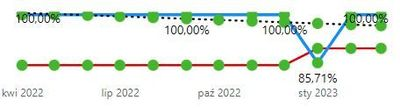cancel
Showing results for
Did you mean:

Fabric is Generally Available. Browse Fabric Presentations. Work towards your Fabric certification with the Cloud Skills Challenge.Helper I

## Trend line (Linear regresion) not working for a graph with "Year" axis

Hello,

I'm using below DAX code to create a trend line:

``````%8D closed on time 12M TREND =
VAR Known =
FILTER (
SELECTCOLUMNS (
ALLSELECTED ( 'COQ Calendar'[WC_Month] ),
"Known[X]", 'COQ Calendar'[WC_Month],
"Known[Y]", [%8D closed on time12M_v2]
),
AND (
NOT ( ISBLANK ( Known[X] ) ),
NOT ( ISBLANK ( Known[Y] ) )
)
)
VAR Count_Items =
COUNTROWS ( Known )
VAR Sum_X =
SUMX ( Known, Known[X] )
VAR Sum_X2 =
SUMX ( Known, Known[X] ^ 2 )
VAR Sum_Y =
SUMX ( Known, Known[Y] )
VAR Sum_XY =
SUMX ( Known, Known[X] * Known[Y] )
VAR Average_X =
AVERAGEX ( Known, Known[X] )
VAR Average_Y =
AVERAGEX ( Known, Known[Y] )
VAR Slope =
DIVIDE (
Count_Items * Sum_XY - Sum_X * Sum_Y,
Count_Items * Sum_X2 - Sum_X ^ 2
)
VAR Intercept =
Average_Y - Slope * Average_X

VAR MaxDate =
MAX ( 'DATE_DUP'[WC_Month] )
VAR MinDate =
DATE ( YEAR ( MaxDate ), MONTH ( MaxDate ) - 12, DAY ( MaxDate ) )

RETURN
CALCULATE(
SUMX (
DISTINCT ( 'COQ Calendar'[WC_Month] ),
Intercept + Slope * 'COQ Calendar'[WC_Month]
),
FILTER ( 'COQ Calendar', 'COQ Calendar'[WC_Month] > MinDate && 'COQ Calendar'[WC_Month] <= MaxDate )
)``````

It is working for graphs where X axis is created as month & year like below:I'm trying to modify the same code to use it for graph where X axis is built only as Years but it is not working:

``````AVG 8D closed on time TREND =
VAR Known =
FILTER (
SELECTCOLUMNS (
ALLSELECTED ( 'COQ Calendar'[WC_Month] ),
"Known[X]", 'COQ Calendar'[WC_Month],
"Known[Y]", SUMX('8D Log','8D Log'[Over 10 Days])/COUNTROWS('8D Log')
),
AND (
NOT ( ISBLANK ( Known[X] ) ),
NOT ( ISBLANK ( Known[Y] ) )
)
)
VAR Count_Items =
COUNTROWS ( Known )
VAR Sum_X =
SUMX ( Known, Known[X] )
VAR Sum_X2 =
SUMX ( Known, Known[X] ^ 2 )
VAR Sum_Y =
SUMX ( Known, Known[Y] )
VAR Sum_XY =
SUMX ( Known, Known[X] * Known[Y] )
VAR Average_X =
AVERAGEX ( Known, Known[X] )
VAR Average_Y =
AVERAGEX ( Known, Known[Y] )
VAR Slope =
DIVIDE (
Count_Items * Sum_XY - Sum_X * Sum_Y,
Count_Items * Sum_X2 - Sum_X ^ 2
)
VAR Intercept =
Average_Y - Slope * Average_X

VAR MaxDate =
MAX ( 'DATE_DUP'[WC_Month] )
VAR MinDate =
DATE ( YEAR ( MaxDate ), MONTH ( MaxDate ) - 12, DAY ( MaxDate ) )

RETURN
CALCULATE (
SUMX (
DISTINCT ( 'COQ Calendar'[WC_Month] ),
Intercept + Slope * 'COQ Calendar'[WC_Month]
),
FILTER ( 'COQ Calendar', 'COQ Calendar'[WC_Month] > MinDate && 'COQ Calendar'[WC_Month] <= MaxDate )
)``````

Result of above code. AS you can see Trend results (orange line) is much higher than KPI result:0 REPLIES 0Announcements#### Power BI Monthly Update - November 2023

Check out the November 2023 Power BI update to learn about new features.#### The largest Power BI and Fabric virtual conference

130+ sessions, 130+ speakers, Product managers, MVPs, and experts. All about Power BI and Fabric. Attend online or watch the recordings.Top Solution Authors
Top Kudoed Authors
Users online (7,099)